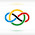### Birthday!

Today (March 18th) is my birthday! I was born on March 18th, 2009.

Thanks to my parents for supporting me in my journey so much :)
I probably would've given up a long time back if it wasn't for them.

Now it's time for 2009 IMO SL #18, which is 2009 IMO SL #G3.

Let $ABC$ be a triangle. The incircle of $ABC$ touches the sides $AB$ and $AC$ at the points $Z$ and $Y$, respectively. Let $G$ be the point where the lines $BY$ and $CZ$ meet, and let $R$ and $S$ be points such that the two quadrilaterals $BCYR$ and $BCSZ$ are parallelogram. Prove that $GR=GS$.

Let the incircle touch $\overline{BC}$ at $X$ and let the $A$-excircle $\omega_A$ touch $\overline{BC}$ at $X'$ and $\overline{AC}$ at $Y'$. Denote $\omega_R$ and $\omega_S$ as the circles centered at $R$ and $S$ respectively, both with radius $0$. Notice that $BR=CY=CX=BX'$ and $YR=BC=AY'-AY=YY'$, so we have that $\overline{BY}$ is the radical axis of $\omega_A$ and $\omega_R$. Similarly, $\overline{CZ}$ is the radical axis of $\omega_A$ and $\omega_S$. Using both of these, we know that the intersection of $\overline{CZ}$ and $\overline{BY}$, namely $G$, is the radical center of $\omega_A$, $\omega_R$, $\omega_S$ and therefore equidistant from $R$ and $S$, as desired. $\square$

1.Anonymous3/18/2022

happy birthday!!

wait...
so you're 13??

i always thought you were like 16 or 17

wow
you're doing amazing work at such a young age
keep it up man

2.Anonymous3/18/2022

happy birthday :)
I like how you're always giving credit to your parents!

3.Anonymous3/20/2022

Well, well, well.

You have done an marvelous job with your work. I wouldn't be so surprised if you were older. Let alone doing these level of problems at this age, but the fact that you're also helping people with this just makes me speechless. Also like @Anonymous said, I really love how modest you are.

Best of luck, buddy!

4.Thank you everyone :D